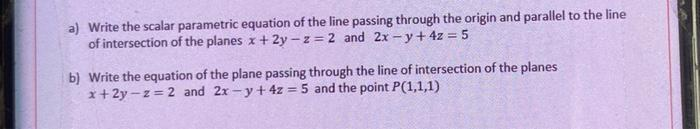# Question a) Write the scalar parametric equation of the line passing through the origin and parallel to the line of intersection of the planes x +2y - 2 = 2 and 2x - y + 4z = 5 b) Write the equation of the plane passing through the line of intersection of the planes x+2y-z= 2 and 2x -y + 4z = 5 and the point P(1,1,1)